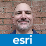# Check if Polygons are equal in SDE

2837
9
01-19-2016 02:06 AMNew Contributor III

Hello,

I have a problem with comparing polygon objects in an (Oracle)SDE.

I have to compare two polygon feature classes that contain the same data from different points in time.

Featureclass "A" contains the "official" polygons from a local government and is delievered to us on a 3-month basis.

Featureclass "B" contains the same set of polygons, but some of them may have been edited by our client.

The total amount of polygons is always the same and they share one ID so that you can always find the "partner" objects in the two sets.

The job is to find all the polygons that have geometric differences between the two sets.

This could be done with ST_Equals or ST_Relate or ST_SymmetricDiff and I tried all of them.

The Problem:

The featureclass that was edited by my client has been processed in a workflow that includes some topology operations.

During that topology geoprocessing ArcGIS inserts additional vertices in nearly every polygon to be able to conduct the operations.

See the part "Note" at ArcGIS Help (10.2, 10.2.1, and 10.2.2)

Those vertices don't change the geometry of the polygon because they are inserted in the middle of straight border lines.

If you compare the sets "A" and "B" with ST_Equals or ST_Relate or ST_SymmetricDiff after one of them has been "treated" by the topology geoprocessing, there are thousands of "differences" although the polygons aren't really changed but only contain additional vertices.

Is there a way to compare polygons while ignoring those additional vertices?

One limitation: It needs to be done within an SQL statement on the SDE.

Thanks for any Idea!

Stefan

Tags (3)
9 RepliesbyEsri Esteemed Contributor

Not an expert on ST_Geometry opertaionss, but what happens if you use a ST_Union—Help | ArcGIS for Desktop and check for equivalence of the ID fields in the output? Those records that don't match will represent the changes...New Contributor III

Hello,

can you elaborate on this?

I don't know how to use that in this case.

Thanks,

StefanRegular Contributor II

So the "Find Identical" tool in ArcGIS is not an option?New Contributor III

Hi,

sadly this is not for us poor "ArcGIS Standard" guys...

Also I am searching for a solution that can be used within an SQL Statement.

Otherwise I would have to make big changes to the already implemented workflow.

Thanks anyway,

StefanRegular Contributor III

Try the code by @Bruce Harold. This code compares two feature classes and produces three files adds, deletes, no change.New Contributor III

Thanks,

I will test this script.

But I would rather have something that can be used within a SQL statement.

Greetings,

StefanbyEsri Contributor

Which version of the SDE/st_shapelib are you using? If I use a WKT example like you mentioned, where there is an extra coordinate on the line between two original points, ST_Equals resolves to true.

`select SDE.ST_EQUALS(sde.st_geometry('POLYGON ((0 0, 2 0, 2 1, 2 2, 0 2, 0 0))', 0), sde.st_geometry('POLYGON ((0 0, 2 0, 2 2, 0 2, 0 0))', 0)) FROM DUAL;`

Could you provide the WKT of the example you have?New Contributor III

Hello,

the ST_EQUALS works in your example because the additional vertex is absolutely exact in a straight line with the corner points.

Yet in a "real-world" example, where the lines are not along the coordinate axis and the extra vertex is a tiny bit out of the mathematical straigt line (for example due to rounding) this tool 'fails'.

Try this:

select SDE.ST_EQUALS(

sde.st_geometry('POLYGON ((0 0, 2 0, 2.0001 1, 2 2, 0 2, 0 0))', 0),

sde.st_geometry('POLYGON ((0 0, 2 0, 2 2, 0 2, 0 0))', 0)) FROM DUAL;

Greetings,

StefanbyEsri Esteemed Contributor

Equivalence usually has a very specific meaning when it comes to geometry:

• Same topology type
• Same number of vertices
• Same vertex values

You are not looking for an "equal" geometry, but something quite a bit more elastic.  The answer could be to use SDE.ST_DIFFERENCE to "subtract" one geometry from the other, and test that for being non-empty.

Assuming two tables:

table1:

A|POLYGON ((0 0, 0 10, 10 10, 10 0, 0 0))

B|POLYGON ((0 10, 10 10, 10 0, 0 0, 0 10))

C|POLYGON ((10 10, 10 0, 0 0, 0 10, 10 10))

table2:

A|POLYGON ((0 0, 0 10, 10 10, 10 0, 0 0))

B|POLYGON ((0 10, 5 10, 10 10, 10 5, 10 0, 5 0, 0 0, 0 5, 0 10))

C|POLYGON ((0 0, 5 10, 10 0, 0 0))

`SQL> select sde.st_isempty(sde.st_difference(a.shape,b.shape))  2  from table1 a, table2 b  3  where a.tid = b.tid;SDE.ST_ISEMPTY(SDE.ST_DIFFERENCE(A.SHAPE,B.SHAPE))--------------------------------------------------                                                 1                                                 1                                                 0`

Curiously, the Oracle implementation of ST_Equals produces that "forgiving" model

`SQL>  select sde.st_equals(a.shape,b.shape)  2   from table1 a, table2 b  3   where a.tid = b.tid;SDE.ST_EQUALS(A.SHAPE,B.SHAPE)------------------------------                             1                             1                             0`

- V